• Sharebar

## Fibonacci method in Forex

Straight to the point:

Fibonacci Retracement Levels are:
0.382, 0.500, 0.618 — three the most important levels
Fibonacci retracement levels are used as support and resistance levels.

Fibonacci Extension Levels are:

0.618, 1.000, 1.618 — three the most important levels
Fibonacci extension levels are used as profit taking levels.

So, what we will learn today is how to apply Fibonacci tool and how to interpret results that we see on the screen.

To set up Fibonacci on the chart we need to find out:
1. Is it uptrend or downtrend?
2. Highest and lowest swings in the chart formation (A, B points).
And go with the trend!

So, click on Fibonacci tool from trading platform that you use. Now, as shown on the Figure 1: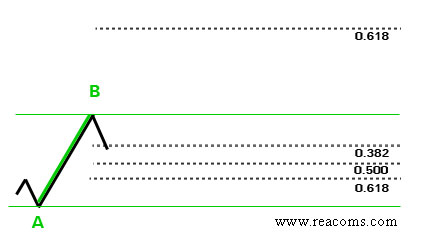We have an uptrend. A — our lowest swing, B — our highest swing. So, we will look to BUY some lots at the good lowest price and go up with the trend.

Click on A and drag your cursor to B,  click. There you go! You must see different lines appeared on your chart. Those lines are called Fibonacci Retracement and Extension Levels.

So, what we are expecting is next: the price should retrace (go down) from point B to some point C, and then continue up in the direction of the trend.

Those three dotted lines (0.618, 0.500, 0.382) at the bottom on our picture shows three Fibonacci retracement levels where we expect the price to take a U-turn and go up again. There we will place our BUY order.

The best situation would be to buy at the lowest level — 0.618 — point C. And on practice the price usually gives us this chance. However, 0.500 is also a good level to place a BUY order.

Well, let’s take a look at the progress.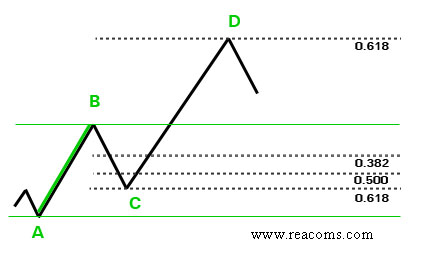The price has successfully reached the lowest 0.618 point and made a U-turn.
So, now when we have our BUY order placed at desired point C, we would like to set some targets to take our profit in the future. For profit taking levels we use Fibonacci extension levels (0.618, 1.000, 1.618). The most common is 0.618 extension level, but when the price shows good potential to reach next 1.000 or even 1.618 level, you can leave your trade to get that target too.

We will choose 0.618 extension level as our profit target, and according to Figure 2, D is our point for taking profit.

Important note: in this Fibonacci tutorial 0.618 extension level (as well as 1.000, 1.618 levels) are calculated in relation to the point B, which means that B point represents a 0% extension.

Some Forex traders like to start counting from point A, then the distance from A to B would be already 100% of the price move. Thus moving further from B would be 1xx.x %.

For example: looking at the last picture, if to start counting from point A, then point D would be a 1.618 Fibonacci extension level or a 161.8% of the price move.

Now let’s have a look at a real forex chart.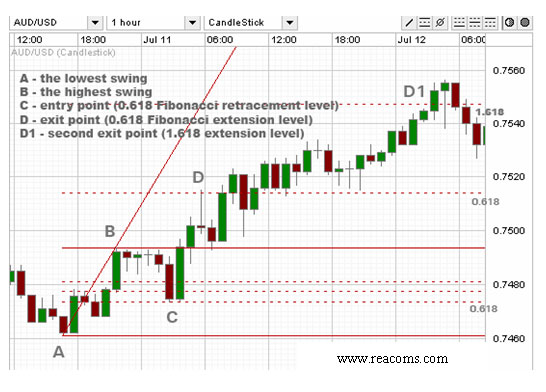Same steps will also apply to downtrend price movement.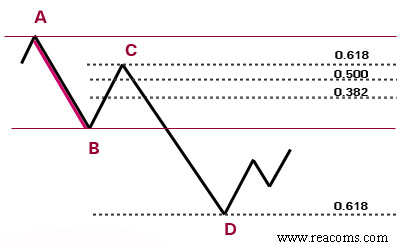A little bit of theory:
Leonardo Fibonacci is a founder of a simple series of numbers related to the natural proportions of things in the universe. Fibonacci numbers  create ratios that arise from the following number series: 0, 1, 1, 2, 3, 5, 8, 13, 21, 34, 55, 89, 144: Calculated this way: 0+1=1, 1+1=2, 1+2=3 and so forth.

The ratio of any number in relevance to the next higher number is 0.618. E.g. 55/89=0.618.

There is no need to perform calculation each time you get into a trade, your trading platform is going to do it for you.

## How to calculate Fibonacci retracement and extension levels

Three most used Fibonacci retracement levels are 0.382 or 38.2%, 0.500 (50%) and 0.618 (61.8%).

Three most used Fibonacci extension levels are 0.618, 1.000 and 1.618. Also 1.382 extension can be applied as well.

Let’s take a look at the next picture: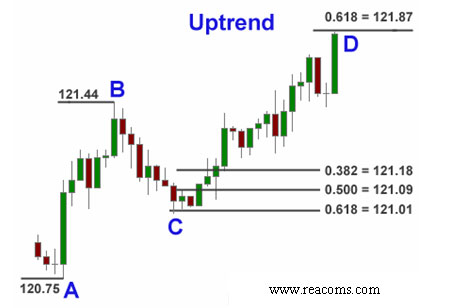In the example above we are in the uptrend. Lowest swing — point A — is 120.75;

highest swing — point B — 121.44.

To calculate retracement levels and enter Long at some point C we do next:

Calculations for Uptrend and Buy order:

B — A = ?
121.44 — 120.75 = 0.69

0.382 (38.2%) retracement = 121.44 — 0.69 x 0.382 = 121.18
0.500 (50.0%) retracement = 121.44 — 0.69 x 0.500 = 121.09

0.618 (61.8%) retracement = 121.44 — 0.69 x 0.618 = 121.01

Fibonacci retracement levels formula for an uptrend:

C = B — (B — A) x N%

Now we need to calculate extension levels:

0.618 (61.8% ) extension = 121.44 + 0.69 x 0.618 = 121.87
1.000 (100.0%) extension = 121.44 + 0.69 x 1.000 = 122.13

1.382 (138.2%) extension = 121.44 + 0.69 x 1.382 = 122.39
1.618 (161.8%) extension = 121.44 + 0.69 x 1.618 = 122.56

Fibonacci extension levels formula for an uptrend:

D = B + (B — A) x N%

Our next example is downtrend.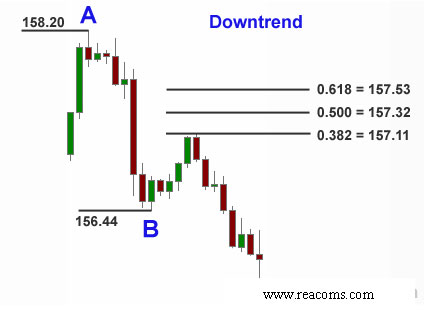Highest swing — point A — is 158.20; lowest swing — point B — is 156.44.

Calculations for downtrend and Sell order:

A — B = ?
158.20 — 156.44 = 1.76

Because of the downtrend we need to add to the lowest point B to find retracement.

0.382 (38.2%) retracement = 156.44 + 1.76 x 0.382 = 157.53
0.500 (50.0%) retracement = 156.44 + 1.76 x 0.500 = 157.32
0.618 (61.8%) retracement = 156.44 + 1.76 x 0.618 = 157.11

Fibonacci retracement levels formula for downtrend:

C = B + (A — B) x N%

Now let’s find Fibonacci extension levels (downtrend):

0.618 (61.8%) extension = 156.44 — 1.76 x 0.618 = 155.35
1.000 (100%) extension = 156.44 — 1.76 x 1.000 = 154.68
1.382 (138.2%) extension = 156.44 — 1.76 x 1.382 = 154.01

1.618 (161.8%) extension = 156.44 — 1.76 x 1.618 = 153.59

Fibonacci extension levels formula for downtrend:

D = B — (A — B) x N%